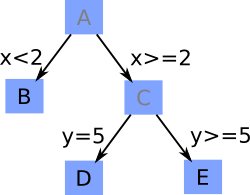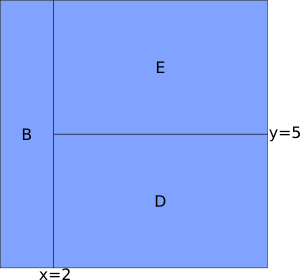### Building a Tree

A decision tree splits the input data into regions by choosing one variable at a time on which to split the data. The splits are hierarchical, so a new split subdivides a previously created region. The simplest situation is a binary split, where only two regions are created from an input region. An interval variable is split by whether the region is less than or is greater than or equal to the split value. Nominal values are collected into two groups.

These hierarchical splits form a tree: the splits are represented by the tree nodes, and the resulting regions are represented by the leaves. Figure 13.3 shows an illustration of a tree and how the space is partitioned by it. The left diagram shows the tree (subdivided region letters are shaded). The splits occur at the tree nodes, and the leaves are the final regions of the input space. The right diagram shows how the input space is partitioned by the tree. The original data set is the region A, which does not appear on the right. Region A is split into regions B and C by the interval variable X. Region C is subdivided again, this time by the variable Y, into regions D and E. Because the largest number of splits that occur in a path from the top of the tree to the bottommost region is two, the depth of this example tree is two.

Figure 13.3: Conceptual Drawing of a Decision Tree

 Tree Partitioned Input Space